# Selina Solutions Concise Maths Class 10 Chapter 13 Section and Mid-Point Formula Exercise 13(A)

Problems based on using section formula and finding the points of trisection are majorly covered in this exercise. Students can refer to the Selina Solutions for Class 10 Maths for knowing the correct procedures to solve problems. It can also be used for clarifying doubts quickly at any time. All the solutions are as per the latest ICSE examination patterns. The Concise Selina Solutions for Class 10 Maths Chapter 13 Section and Mid-Point Formula Exercise 13(A) PDF can be accessed in the links given below.

## Selina Solutions Concise Maths Class 10 Chapter 13 Section and Mid-Point Formula Exercise 13(A) Download PDF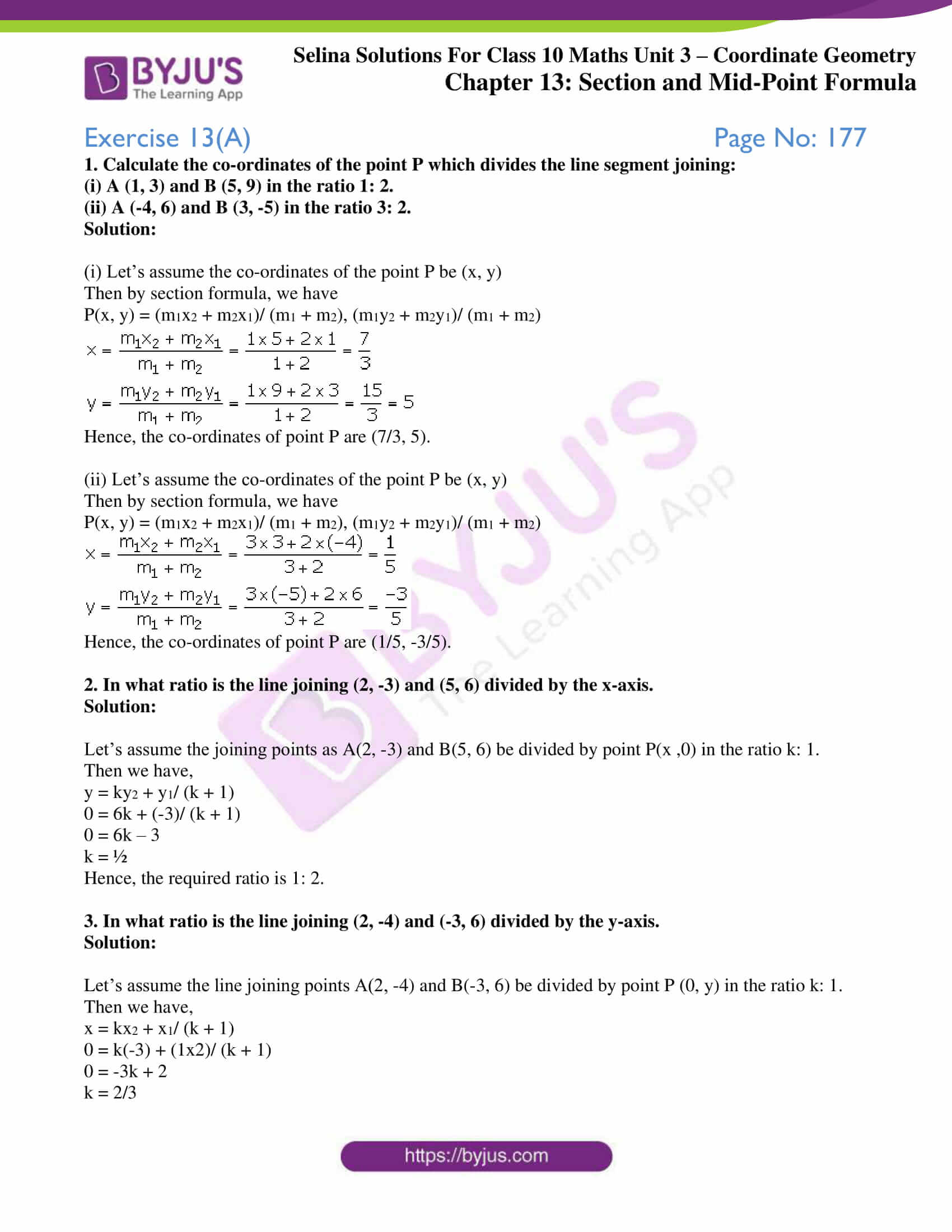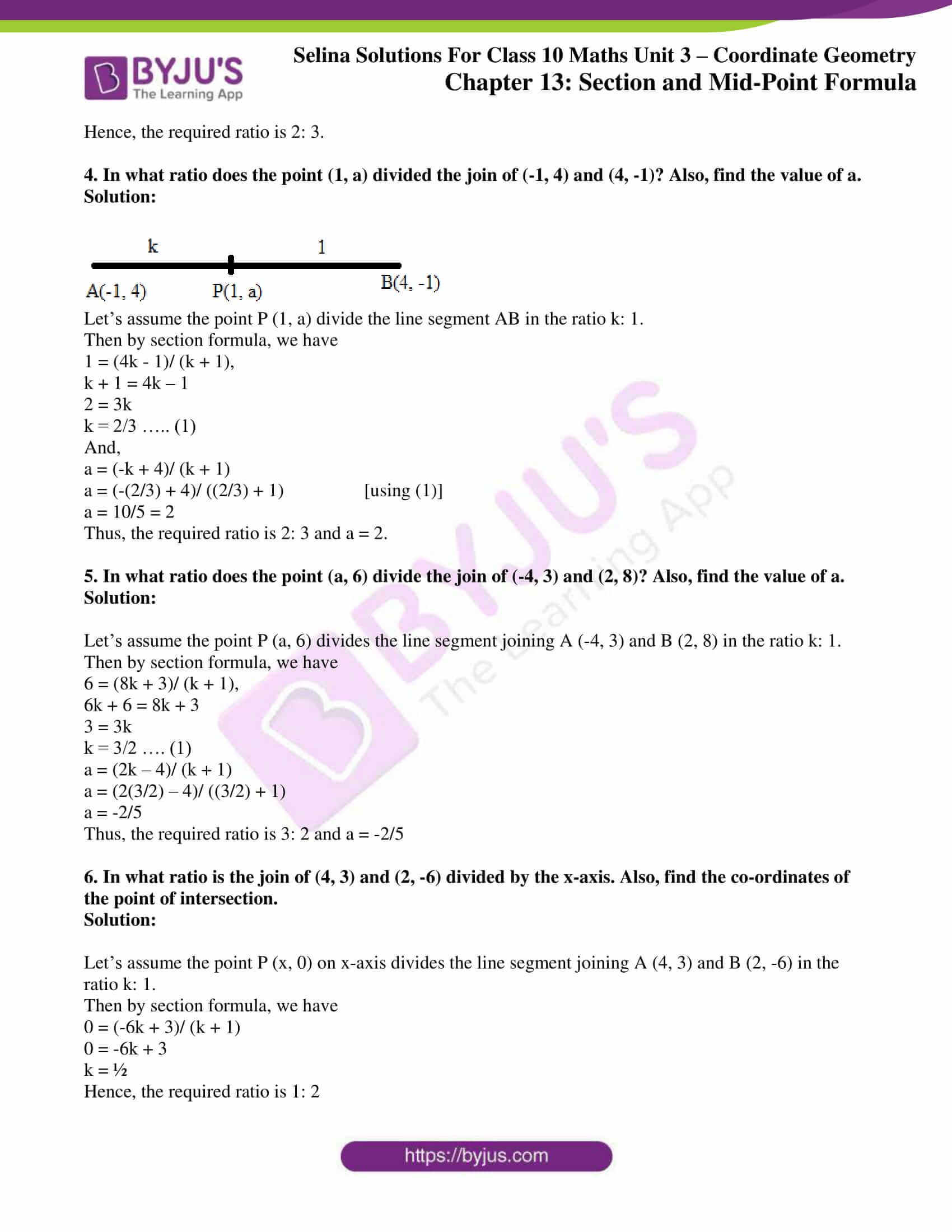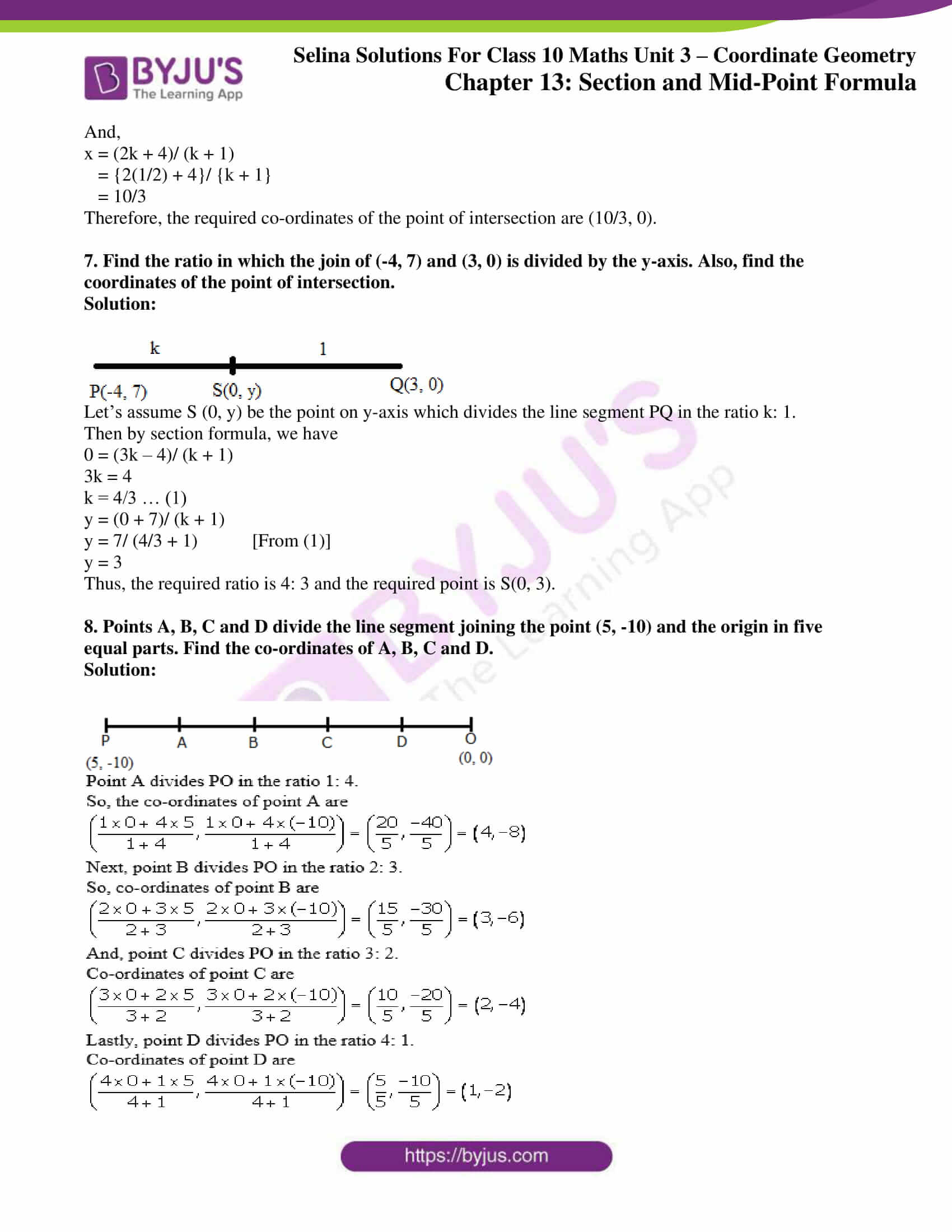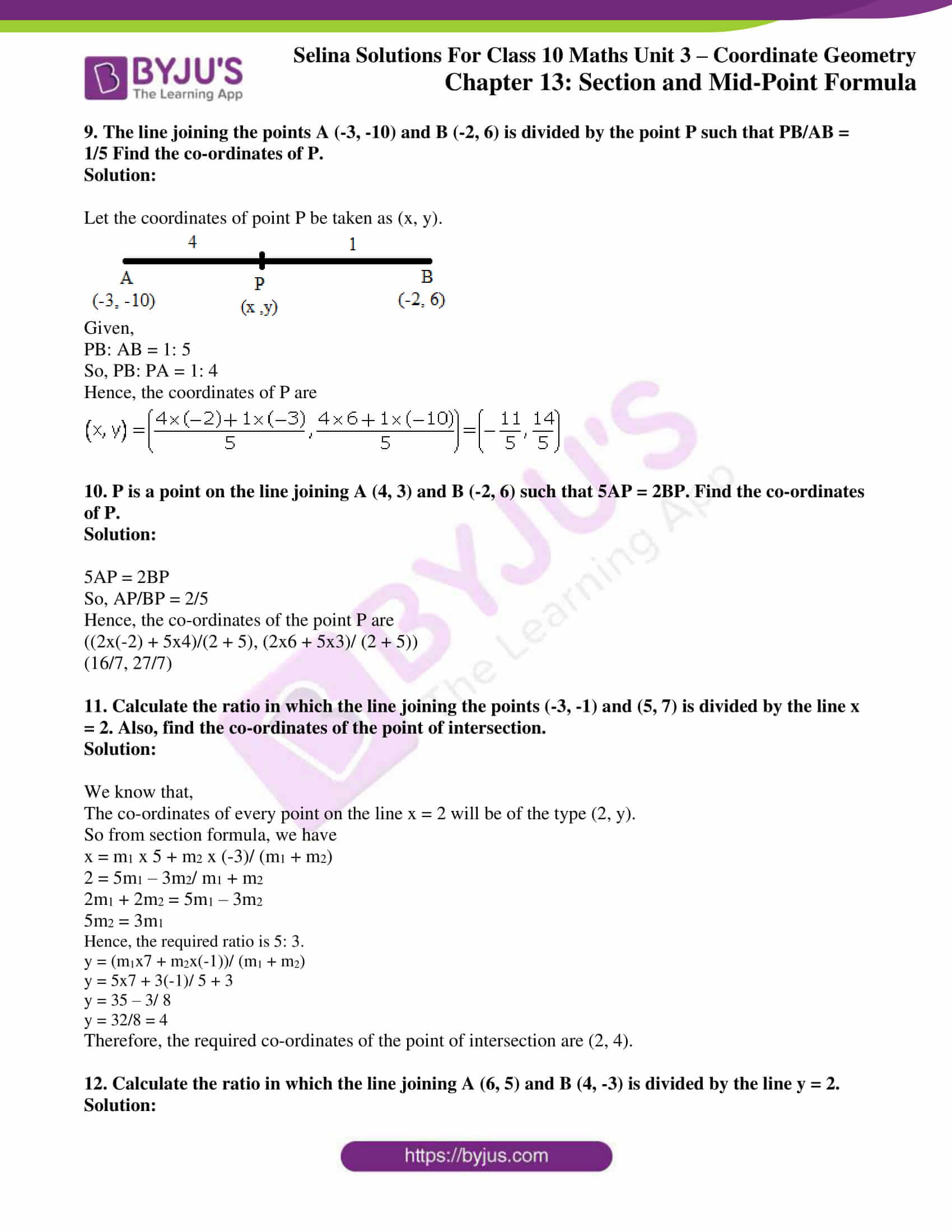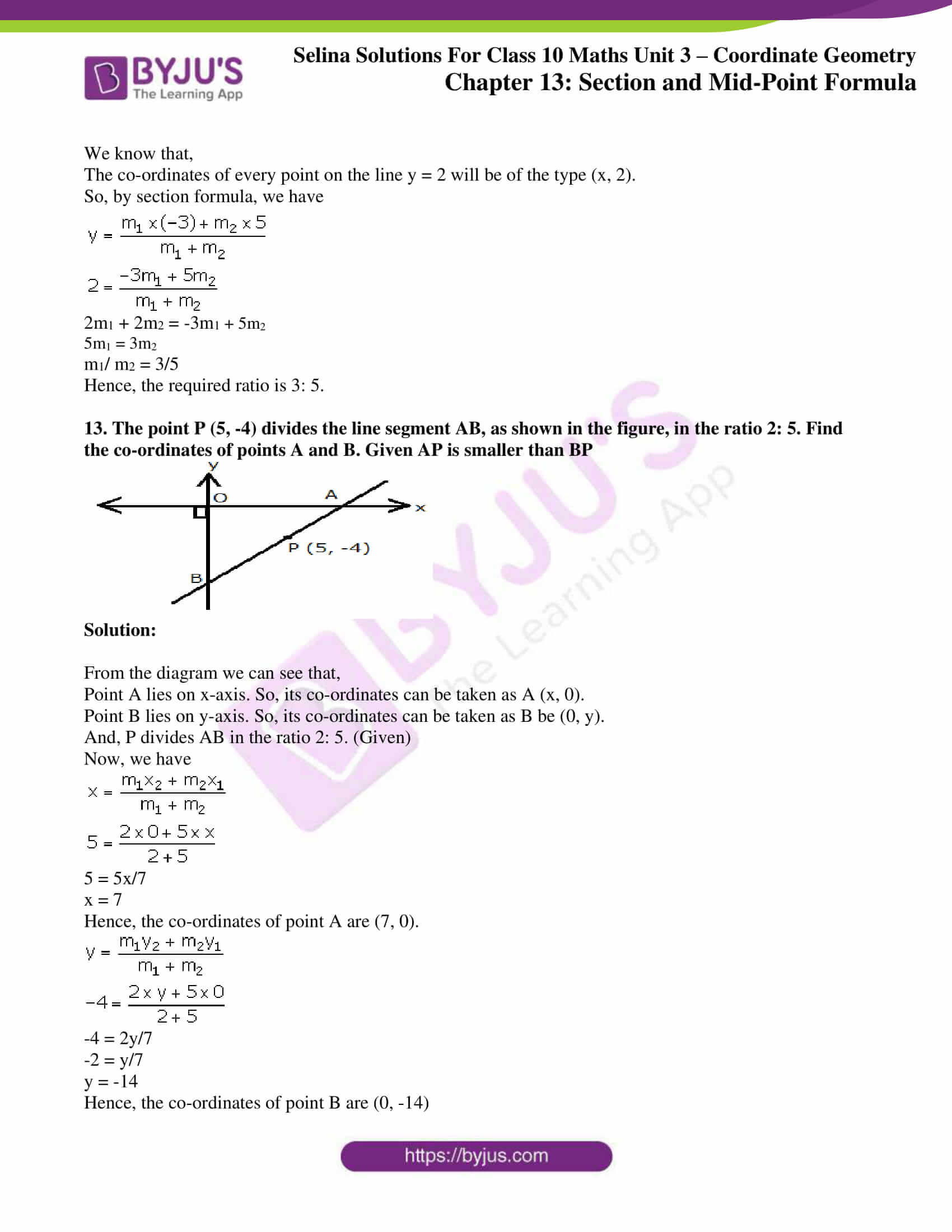### Access Selina Solutions Concise Maths Class 10 Chapter 13 Section and Mid-Point Formula Exercise 13(A)

1. Calculate the co-ordinates of the point P which divides the line segment joining:

(i) A (1, 3) and B (5, 9) in the ratio 1: 2.

(ii) A (-4, 6) and B (3, -5) in the ratio 3: 2.

Solution:

(i) Let’s assume the co-ordinates of the point P be (x, y)

Then by section formula, we have

P(x, y) = (m1x2 + m2x1)/ (m1 + m2), (m1y2 + m2y1)/ (m1 + m2)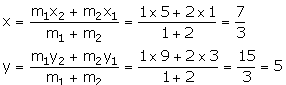Hence, the co-ordinates of point P are (7/3, 5).

(ii) Let’s assume the co-ordinates of the point P be (x, y)

Then by section formula, we have

P(x, y) = (m1x2 + m2x1)/ (m1 + m2), (m1y2 + m2y1)/ (m1 + m2)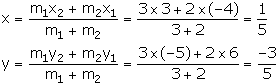Hence, the co-ordinates of point P are (1/5, -3/5).

2. In what ratio is the line joining (2, -3) and (5, 6) divided by the x-axis.

Solution:

Let’s assume the joining points as A(2, -3) and B(5, 6) be divided by point P(x ,0) in the ratio k: 1.

Then we have,

y = ky2 + y1/ (k + 1)

0 = 6k + (-3)/ (k + 1)

0 = 6k – 3

k = ½

Hence, the required ratio is 1: 2.

3. In what ratio is the line joining (2, -4) and (-3, 6) divided by the y-axis.

Solution:

Let’s assume the line joining points A(2, -4) and B(-3, 6) be divided by point P (0, y) in the ratio k: 1.

Then we have,

x = kx2 + x1/ (k + 1)

0 = k(-3) + (1×2)/ (k + 1)

0 = -3k + 2

k = 2/3

Hence, the required ratio is 2: 3.

4. In what ratio does the point (1, a) divided the join of (-1, 4) and (4, -1)? Also, find the value of a.

Solution: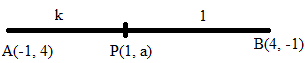Let’s assume the point P (1, a) divide the line segment AB in the ratio k: 1.

Then by section formula, we have

1 = (4k – 1)/ (k + 1),

k + 1 = 4k – 1

2 = 3k

k = 2/3 ….. (1)

And,

a = (-k + 4)/ (k + 1)

a = (-(2/3) + 4)/ ((2/3) + 1) [using (1)]

a = 10/5 = 2

Thus, the required ratio is 2: 3 and a = 2.

5. In what ratio does the point (a, 6) divide the join of (-4, 3) and (2, 8)? Also, find the value of a.

Solution:

Let’s assume the point P (a, 6) divides the line segment joining A (-4, 3) and B (2, 8) in the ratio k: 1.

Then by section formula, we have

6 = (8k + 3)/ (k + 1),

6k + 6 = 8k + 3

3 = 3k

k = 3/2 …. (1)

a = (2k – 4)/ (k + 1)

a = (2(3/2) – 4)/ ((3/2) + 1)

a = -2/5

Thus, the required ratio is 3: 2 and a = -2/5

6. In what ratio is the join of (4, 3) and (2, -6) divided by the x-axis. Also, find the co-ordinates of the point of intersection.

Solution:

Let’s assume the point P (x, 0) on x-axis divides the line segment joining A (4, 3) and B (2, -6) in the ratio k: 1.

Then by section formula, we have

0 = (-6k + 3)/ (k + 1)

0 = -6k + 3

k = ½

Hence, the required ratio is 1: 2

And,

x = (2k + 4)/ (k + 1)

= {2(1/2) + 4}/ {k + 1}

= 10/3

Therefore, the required co-ordinates of the point of intersection are (10/3, 0).

7. Find the ratio in which the join of (-4, 7) and (3, 0) is divided by the y-axis. Also, find the coordinates of the point of intersection.

Solution: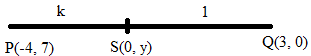Let’s assume S (0, y) be the point on y-axis which divides the line segment PQ in the ratio k: 1.

Then by section formula, we have

0 = (3k – 4)/ (k + 1)

3k = 4

k = 4/3 … (1)

y = (0 + 7)/ (k + 1)

y = 7/ (4/3 + 1) [From (1)]

y = 3

Thus, the required ratio is 4: 3 and the required point is S(0, 3).

8. Points A, B, C and D divide the line segment joining the point (5, -10) and the origin in five equal parts. Find the co-ordinates of A, B, C and D.

Solution: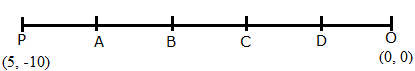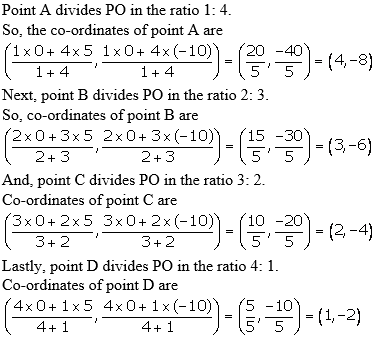9. The line joining the points A (-3, -10) and B (-2, 6) is divided by the point P such that PB/AB = 1/5 Find the co-ordinates of P.

Solution:

Let the coordinates of point P be taken as (x, y).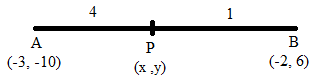Given,

PB: AB = 1: 5

So, PB: PA = 1: 4

Hence, the coordinates of P are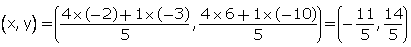10. P is a point on the line joining A (4, 3) and B (-2, 6) such that 5AP = 2BP. Find the co-ordinates of P.

Solution:

5AP = 2BP

So, AP/BP = 2/5

Hence, the co-ordinates of the point P are

((2x(-2) + 5×4)/(2 + 5), (2×6 + 5×3)/ (2 + 5))

(16/7, 27/7)

11. Calculate the ratio in which the line joining the points (-3, -1) and (5, 7) is divided by the line x = 2. Also, find the co-ordinates of the point of intersection.

Solution:

We know that,

The co-ordinates of every point on the line x = 2 will be of the type (2, y).

So from section formula, we have

x = m1 x 5 + m2 x (-3)/ (m1 + m2)

2 = 5m1 – 3m2/ m1 + m2

2m1 + 2m2 = 5m1 – 3m2

5m2 = 3m1

Hence, the required ratio is 5: 3.

y = (m1x7 + m2x(-1))/ (m1 + m2)

y = 5×7 + 3(-1)/ 5 + 3

y = 35 – 3/ 8

y = 32/8 = 4

Therefore, the required co-ordinates of the point of intersection are (2, 4).

12. Calculate the ratio in which the line joining A (6, 5) and B (4, -3) is divided by the line y = 2.

Solution:

We know that,

The co-ordinates of every point on the line y = 2 will be of the type (x, 2).

So, by section formula, we have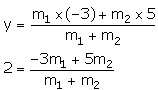2m1 + 2m2 = -3m1 + 5m2

5m1 = 3m2

m1/ m2 = 3/5

Hence, the required ratio is 3: 5.

13. The point P (5, -4) divides the line segment AB, as shown in the figure, in the ratio 2: 5. Find the co-ordinates of points A and B. Given AP is smaller than BP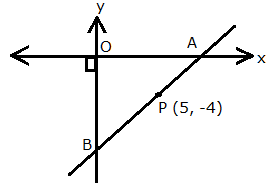Solution:

From the diagram we can see that,

Point A lies on x-axis. So, its co-ordinates can be taken as A (x, 0).

Point B lies on y-axis. So, its co-ordinates can be taken as B be (0, y).

And, P divides AB in the ratio 2: 5. (Given)

Now, we have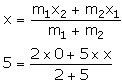5 = 5x/7

x = 7

Hence, the co-ordinates of point A are (7, 0).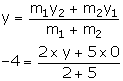-4 = 2y/7

-2 = y/7

y = -14

Hence, the co-ordinates of point B are (0, -14)